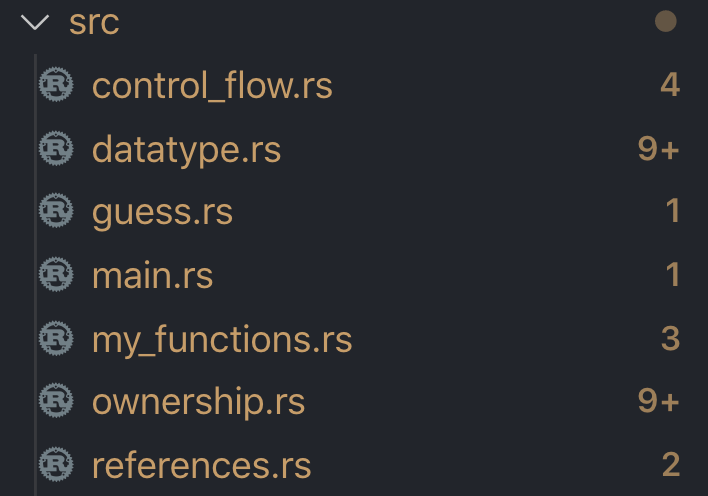#DAY 10
0

## Module

Rust 的 entry point 是 main 這個函式，而筆者在寫的時候其實就已經把函式都拆開來在不同的檔案裡面了，所以我的函式名稱都不是用 main 來命名會跟官方的範例有一點出入。這邊我就直接帶各位看一下我的專案結構##### 檔案名稱：my_functions.rs
``````fn hello_world() {
println!("Hello World");
}
``````

##### 檔案名稱 main.rs
``````mod my_functions;

fn main() {
my_functions::hello_world();
}
``````

##### 檔案名稱 main.rs
``````mod my_functions;

fn main() {
my_functions::hello_world();
^^^^^^^^^^^ function `hello_world` is private
}
``````

##### 檔案名稱：my_functions.rs
``````pub fn hello_world() {
println!("Hello World");
}
``````

• mod 宣告一個 module
• pub 輸出
• use 載入

## 函式參數

``````fn fn_with_parameters(x: i32, y: i32) {
println!("The value of x is: {}", x);
println!("The value of y is: {}", y);
}
``````

``````fn main() {
fn_with_parameters(5, 5);
// The value of x is: 5
// The value of y is: 5
}
``````

``````fn main() {
fn_with_parameters('5', 5);
^^^ expected i32, found char
// The value of x is: 5
// The value of y is: 5
}
``````

`Note: 函式參數必須給他指定的型態，如果傳進來的參數型態錯誤時就會直接報錯。`

## expression vs statement

Rust 的一個重要概念就是他是 expression-based language ，而 expression 意思就是說他一定會有返回的值反之 statement 則無返回值。這樣設計的目的可以讓我們的程式可以很好的被組合來使用也非常符合 functional programing 的概念。

``````fn expression_based() {
let foo = if 1 == 0 {
"bar"
} else {
"baz"
};
println!("The value of foo is: {}", foo);
// The value of foo is: baz
}
``````

`Note: 函式是屬於 statement 的別搞錯了喔。`

## 函式包含 expression 和 statement

Rust 的函式中可以包含很多的 expression 或是 statement 剛剛所講的 expression 其實就是 {} 而其都一定會有返回值，但是 statement 則一定不會有返回值，舉個例子

``````fn main() {
let x = (let y = 6);
}
``````

``````let x = y = 1;
``````

`Note: 這是 javascript。`

``````fn statement_test() {
let x = {
let y = 6;
y
};
println!("The value of x is: {}", x);
// The value of x is: 6
}
``````

expression 返回 y 所以 x 也跟 y 一樣為 6，另外一點值得注意的是 expression 的結尾設計上並沒有分號這點要稍加留意囉

## 函式返回值

``````fn five() -> i32 {
5
}
``````

``````fn add(x: i32, y: i32) -> i32 {
x + y
}
``````

``````pub fn add(x: i32, y: i32) -> i32 {
return x + y;
}
``````

## 最後一樣有問題歡迎發問